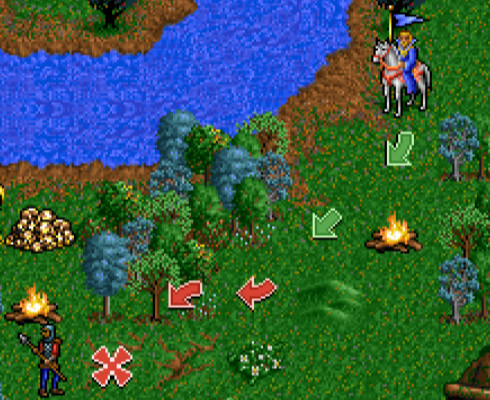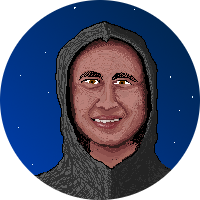/ #mechanics

# Movement: Grid-based

Grid-based movement is a straightforward movement system often used in strategy games, board-games and roguelikes.

At its most basic a grid-based movement system consists of a world defined as a grid and a unit defined as an (x, y) coordinate that can be moved in 8 directions, as shown in the figure below. The most prominent visible contrast with free movement is that the unit moves multiple pixels per move, so for example, if the map tiles are 8x8 pixels, then moving to the right one tile will move the unit 8 pixels to the right. Many games disguise this visual contrast by using animation to gradually move the sprite from its original position to its new location.As the greyed out diagonal arrows suggest, not all games allow diagonal movement so technically there are a few variants of this system:

• Orthogonal: up, down, left and right only.
• Diagonal: This term usually refers to orthogonal plus the diagonal directions being allowed.
• Diagonal only: Only allow movement on diagonals axes, not on orthogonal axes - I don’t think I’ve ever seen this used.

Many games with grid-based movement don’t show the grid, for example here’s a pretty screenshot from Heroes of Might and Magic showing the diagonal movement system with the grid nearly invisible thanks to good tile design.The following demo tries to illustrate the three types of grid-based movement. You can move around with the arrow keys and use X/C to switch between orthogonal, diagonal and diagonal-only modes. Note that for the diagonal modes you have to hold down the arrow keys to move, holding two keys simultaneously if you want to move diagonally:

## Code Example

This section highlights the essential bits of the code for this mechanic.

The full code for the demo above is available at https://gitlab.com/tbs-mechanics/movement_grid_based. I built the demo with PICO-8, so here’s an API Reference which should clarify anything that’s not vanilla Lua.

### Data

As with basic movement, the data needed to represent movement is elementary. A Cartesian coordinate, so `(x,y)` in 2D space, or `(x,y,z)` in 3D space. This time that coordinate refers to a position on the grid, rather than a pixel position on the screen. If you need to store data about positions in the grid, you would typically also model the grid itself as a nested array of grid positions. However, for this demo, I’m using PICO-8’s map system for that, so you don’t see it directly presented in code.

The `speed` variable from basic movement is no longer necessary, as moving multiple tiles with a single input is relatively unusual. Multi-tile-movement would involve indirect controls using AI path-finding algorithms: both topics that I will cover separately (see related mechanics).

The `dx` and `dy` variables (d for delta) are unusual. However, I built the demo with PICO-8, which only supports four directional inputs, so I had to add some extra code to make diagonal movements possible. The `player.delay`, `key_delay` and `clear_delay` are all there for the same reason.

``````function _init()
entities={}

-- Player coordinates
player={}
player.x=64
player.y=64

map_w=64
map_h=64

-- extra bits here to deal with PICO-8's limited inputs
player.dx=0
player.dy=0
player.delay=0

-- in diagonal modes, commit
-- move after 5 frames of input
key_delay=5

-- in diagonal modes, clear
-- if no input for 2 frames
clear_delay=2

-- map initialisation also happens here,
-- but that's out of scope for this demo
end
``````

Also, note the global `map_w` and `map_h` variables for the map width and height. These variables are used in calculations to prevent the character from moving out of the bounds of the world.

### Update Function

For this demo, I have three update functions, one for each movement mode.

For orthogonal movement:

``````function update_simple_orthogonal()
-- delay input slightly to prevent moving too fast
if player.delay > 0 then
player.delay -= 1
return
end

if btn(0) then
-- left
player.x = min(map_w-1, max(0, player.x - 1))
player.delay = key_delay
elseif btn(1) then
-- right
player.x = min(map_w-1, max(0, player.x + 1))
player.delay = key_delay
elseif btn(2) then
-- up
player.y = min(map_h-1, max(0, player.y - 1))
player.delay = key_delay
elseif btn(3) then
-- down
player.y = min(map_h-1, max(0, player.y + 1))
player.delay = key_delay
end
end
``````

Diagonal movement is quite complex due to PICO-8 only having four directional inputs instead of 8. Fundamentally the point is to allow the x-axis and y-axis to change at once:

``````function update_weird_diagonal()
-- delay input slightly to prevent moving too fast
player.delay+=1

-- note if if if, instead of if-else to allow simultaneous inputs
if btn(0) then
-- input left
player.dx -= 1
player.delay = 0
end
if btn(1) then
-- input right
player.dx += 1
player.delay = 0
end
if btn(2) then
-- input up
player.dy -= 1
player.delay = 0
end
if btn(3) then
-- input down
player.dy += 1
player.delay = 0
end

-- clear input after slight delay to avoid ghost inputs from previous moves
if player.delay > clear_delay then
player.dx = 0
player.dy = 0
end

-- key needs to be held for at least delay in diagonal movement mode
if abs(player.dx) > key_delay or abs(player.dy) > key_delay then
if player.dx ~= 0 then
player.x += flr(player.dx / abs(player.dx)) -- +/- 1 (preserve sign)
player.x = min(map_w-1, max(0, player.x)) -- clamp to map area
end

if player.dy~=0 then
player.y+=flr(player.dy/abs(player.dy)) -- +/- 1 (preserve sign)
player.y=min(map_h-1,max(0,player.y)) -- clamp to map area
end

-- clear input and wait for delay before moving again
player.dx=0
player.dy=0
end
end
``````

Finally, diagonal-only movement, basically as for diagonal but only allow the move if BOTH x-axis and y-axis are changing:

``````function update_diagonal_only()
-- delay input slightly to prevent moving too fast
if player.delay > 0 then
player.delay -= 1
return
end

if btn(0) then
-- left
player.x = min(map_w-1, max(0, player.x - 1))
player.delay = key_delay
elseif btn(1) then
-- right
player.x = min(map_w-1, max(0, player.x + 1))
player.delay = key_delay
elseif btn(2) then
-- up
player.y = min(map_h-1, max(0, player.y - 1))
player.delay = key_delay
elseif btn(3) then
-- down
player.y = min(map_h-1, max(0, player.y + 1))
player.delay = key_delay
end

-- clear input after slight delay to avoid ghost inputs from previous moves
if player.delay > clear_delay then
player.dx = 0
player.dy = 0
end

if abs(player.dx) > key_delay or abs(player.dy) > key_delay then
if player.dx ~= 0 and player.dy ~= 0 then
player.x += flr(player.dx / abs(player.dx))
player.y += flr(player.dy / abs(player.dy))
player.x = min(map_w-1, max(0,player.x))
player.y = min(map_h-1, max(0,player.y))
player.delay = 0
end

-- clear input and wait for delay before moving again
player.dx = 0
player.dy = 0
end
end
``````#### Matt Van Der Westhuizen

Back-end service developer at Ubisoft Blue Byte by day - wannabe game designer & developer by night.